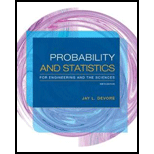# Nonpoint source loads are chemical masses that travel to the main stem of a river and its tributaries in flows that are distributed over relatively long stream reaches, in contrast to those that enter at well-defined and regulated points. The article “Assessing Uncertainty in Mass Balance Calculation of River Nonpoint Source Loads” ( J. of Envir. Engr ., 2008: 247–258) suggested that for a certain time period and location, X 5 nonpoint source load of total dissolved solids could be modeled with a lognormal distribution having mean value 10,281 kg/day/km and a coefficient of variation C V = .40 ( C V = σ X / μ X ) . a. What are the mean value and standard deviation of ln( X )? b. What is the probability that X is at most 15,000 kg/day/km? c. What is the probability that X exceeds its mean value, and why is this probability not .5? d. Is 17,000 the 95th percentile of the distribution?### Probability and Statistics for Eng...

9th Edition
Jay L. Devore
Publisher: Cengage Learning
ISBN: 9781305251809### Probability and Statistics for Eng...

9th Edition
Jay L. Devore
Publisher: Cengage Learning
ISBN: 9781305251809

#### Solutions

Chapter 4.5, Problem 79E
Textbook Problem

## Expert Solution

### Want to see the full answer?

Check out a sample textbook solution.

### Want to see this answer and more?

Experts are waiting 24/7 to provide step-by-step solutions in as fast as 30 minutes!*

*Response times vary by subject and question complexity. Median response time is 34 minutes and may be longer for new subjects.